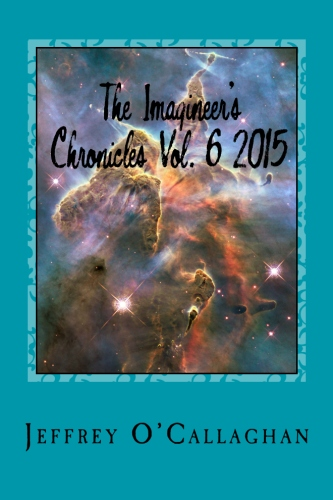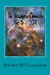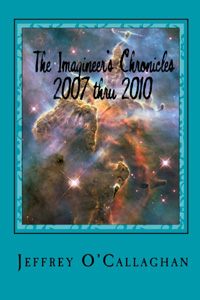## A Relativistic Quantum Mechanics

Quantum mechanistic defines our observable environment only in terms of the probabilistic values associated with Schrödinger’s wave equation.

Many interpret this as meaning a particle and all other objects exists in a world of probabilities and only become connected to the environment when observed.  Additionally it assumes that a particle is distributed or simultaneous exists form one edge of the universe to the other because it tells us there is a probability it can be found anywhere in it.

However it is extremely difficult to define a set of statements which explains how those probabilities can be physically connected to that environment even though it has held up to rigorous and thorough experimental testing.

Yet Einstein gave us a an explanation for this connection in his relativistic formulas for length contraction L = L0((1 – v2/c2))1/2 because it tells us the distance between every point along the trajectory of all forms of energy which are moving at the speed including that associated with the wavefunction is zero for an observer who is outside of its reference frame.  In other words since the energy associated with Schrödinger’s equation which is moving at the speed of light the distance between the each end of the universe for it relative to an outside observer is zero.

However because the probabilities associated with Schrödinger’s equation involve the spatial properties of position, to fully understand the ramifications of that equation to our understanding of quantum mechanics one must transpose it to the spatial equivalent.

Einstein gave us the ability to do this when he defined the geometric properties of space-time in terms of the constant velocity of light and a dynamic balance between mass and energy because that  provided a method of converting a unit of time in a space-time environment of unit of space in four *spatial* dimensions.  Additionally because the velocity of light is constant he also defined a one to one quantitative and qualitative correspondence between his space-time universe and one made up of four *spatial* dimensions.

The fact that one can use Einstein’s equations to qualitatively and quantitatively redefine the curvature in space-time he associated with energy in terms of four *spatial* dimensions is one bases for assuming as was done in the article “Defining energy?” Nov 27, 2007 that all forms of energy can be derived in terms of a spatial displacement in a "surface" of a three-dimensional space manifold with respect to a fourth *spatial* dimension.

However this would allow one to physically the connect the probabilities associated Schrödinger’s equation to our observable environment in terms of a physical or spatial displacement in a "surface" of a three-dimensional space manifold with respect to a fourth *spatial* dimension as was done in the article "Why is energy/mass quantized?" Oct. 4, 2007.

Briefly that article showed that the observable properties of particles can be caused by the formation of a resonant system on a "surface" of a three-dimensional space manifold with respect to fourth "spatial" dimension.  This is because the four conditions required for resonance to occur in a three-dimensional environment, an object, or substance with a natural frequency, a forcing function at the same frequency as the natural frequency, the lack of a damping frequency and the ability for the substance to oscillate spatial would occur in one made up of four.

The existence of four *spatial* dimensions would give a matter wave the ability to oscillate spatially on a "surface" between a third and fourth *spatial* dimension thereby fulfilling one of the requirements for classical resonance to occur.

These oscillations would be caused by an event such as the decay of a subatomic particle or the shifting of an electron in an atomic orbital.  This would force the "surface" of a three-dimensional space manifold with respect to a fourth *spatial* dimension to oscillate with the frequency associated with the energy of that event.

However, the oscillations caused by such an event would serve as forcing function allowing a resonant system or "structure" to be established on a surface of a three-dimensional space manifold.

Yet the classical laws of three-dimensional space tell us the energy of resonant systems can only take on the discontinuous or discreet energies associated with their fundamental or harmonic of their fundamental frequency.

However, these are the similar to the quantum mechanical properties of energy/mass in that they can only take on the discontinuous or discreet energies associated with the fundamental resonate  frequency of space defined by the equation E=hv where "E" equals the energy of a particle "h" equal Planck’s constant "v" equals the frequency of its wave component.

Yet it also allows one to define the physical boundaries of a quantum system in terms of the geometric properties of four *spatial* dimensions.

For example in classical physics, a point on the two-dimensional surface of a piece of paper is confined to that surface.  However, that surface can oscillate up or down with respect to three-dimensional space.

Similarly an object occupying a volume of three-dimensional space would be confined to it however, it could, similar to the surface of the paper oscillate “up” or “down” with respect to a fourth *spatial* dimension.

The confinement of the “upward” and “downward” oscillations of a three-dimension volume with respect to a fourth *spatial* dimension is what defines the spatial boundaries associated with a particle in the article "Why is energy/mass quantized?" Oct. 4, 2007

As mentioned earlier in the article “Defining energy?” Nov 27, 2007 showed all forms of energy can be derived in terms of a spatial displacement in a "surface" of a three-dimensional space manifold with respect to a fourth *spatial* dimension.

However as mentioned earlier assuming the probabilities associated with Schrödinger’s equation are the result of a displacement caused by a matter wave moving on a "surface" of a three-dimension space manifold with respect to a four *spatial* dimension allows one to connect them to the physicality of the observable environment we all live in.

Classical mechanics tell us that due to the continuous properties of the wave energy the article "Why is energy/mass quantized?" Oct. 4, 2007 associated with a quantum system would be distributed throughout the entire "surface" a three-dimensional space manifold with respect to a fourth *spatial* dimension.

For example Classical mechanics tells us that the energy of a vibrating or oscillating ball on a rubber diaphragm would be disturbed over its entire surface while the magnitude of those vibrations would decrease as one move away from the focal point of the oscillations.

Similarly if the assumption that quantum properties of energy/mass are a result of vibrations or oscillations in a "surface" of three-dimensional space caused by matter wave is correct then classical mechanics tell us that those oscillations would be distributed over the entire "surface" three-dimensional space while the magnitude of those vibrations would be greatest at the focal point of the oscillations and decreases as one moves away from it because

However, as was mentioned earlier Einstein in his formula for length contraction L = L0((1 – v2/c2))1/2 tells us that a particle would simultaneously exist everywhere throughout the entire universe because of the fact that wave energy is continuous it would extend to each end of the universe.  Therefore the distance between the each end of the universe relative to an observer outside of that reference frame would be zeroAdditionally because time stops for anything traveling at the speed of light it would appear to exist simultaneously at every point in the universe of an outside observer.

As mentioned earlier the article “Why is energy/mass quantized?” shown a quantum particle is a result of a resonant structure formed on the "surface" of a three-dimensional space manifold with respect to a fourth *spatial* dimension.

Yet Classical Wave Mechanics tells us resonance would most probably occur on the surface of the rubber sheet were the magnitude of the vibrations is greatest and would diminish as one move away from that point.

Similarly a particle would most probably be found were the magnitude of the vibrations in a "surface" of a three-dimensional space manifold is greatest and would diminish as one move away from that point.

In others words one can explain how the probabilities associated with Schrödinger’s equation are connected to our physical world and the fact that particles simultaneously exist everywhere in the universe before it is observed by applying the concepts of Einstein Theory of Relativity to the quantum environment.

It should be remember Einstein’s genius allows us to choose to define a quantum system in either a space-time environment or one consisting of four *spatial* dimension when he defined the geometry of space-time in terms of the constant velocity of light. This interchangeability broadens the environment encompassed by his theories thereby giving us a new perspective on the probabilistic properties of a quantum environment and how they physically connected to our observable universe.

Later Jeff

 Anthology of The Imagineer’s Chronicles Vol. 1 thru 7 2007 thru 2016Ebook \$10.50 The Reality of the Fourth Spatial Dimension  Paperback \$9.77 Ebook    \$6.24 The Imagineer’s Chronicles Vol. 7 — 2016Paperback \$11.25 E-book \$7.25 The Imagineer’s Chronicles Vol. 6 — 2015Paperback \$12.25 E-book \$9.89Paperback \$14.84 Ebook \$9.97 The Imagineer’s Chronicles Vol. 2 — 2011Paperback \$9.24 Ebook \$6.57 The Imagineer’s Chronicles 2007 thru 2010Paperback \$15.43 Ebook \$7.82see more...